# 随机变量的数学特征：均值、方差、协方差、相关系数

2014/10/26 12:08

## 数学期望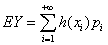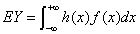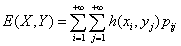### 性质

1、若C是常数，则EC=C
2、设X是一个随机变量，且Y=aX+b，EY=aEX+b
3、
X，Y是两个随机变量，E(X+Y)=EX+EY(注意：这个条件没有要求X，Y独立)
4、若X，Y是两个独立的随机变量，则E(XY)=E(X)E(Y)

## 方差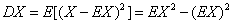### 性质

1、C为常数，D(C)=0;
2、a,b是常数，X是随机变量，D(aX+b)=a^2D(X)
3、X，Y是两个独立的随机变量，D(X+Y)=DX+DY

## 协方差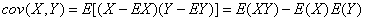### 性质

1、cov(X,Y)=cov(Y,X)
2、对任意实数a,b,c,d,有cov(aX+b,cY+d)=abcov(X,Y)

3、cov(X1+X2,Y)=cov(X1,Y)+cov(X2,Y)

4、若X，Y独立，则cov(X,Y)=0

5、对任意a，b，有D(aX+bY+c)=a^2DX+b^2DY+2abcov(X,Y)

## 相关系数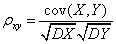### 性质

1、|p|<=1
2、若X，Y相互独立，则Pxy=0，反之不一定成立。
3、|Pxy|=1的充要条件是存在常数a,b,使得P（Y=aX+b）=1,且Pxy=1，当a>0,Pxy=-1，当a<0

## 随机变量X的标准化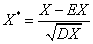0
0 收藏

### 作者的其它热门文章0 评论
0 收藏
0